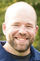General Discussion

## Powershell Question1,306 Views

I need to find the space used by only a select group of volumes.

PS C:\WINDOWS\system32> Get-NcVol | Where-Object { \$_.State -eq 'offline'} |  ( ????)

What command can I use to get the total space used by only the offine volumes?

Thanks

1 ACCEPTED SOLUTION1,292 Views

You got it about 95% of the way there. I would do:

` Get-NcVol | Where-Object {\$_.State -eq "offline"} | select Name, @{l = "TotalSizeGB"; e = {\$([Math]::Round(\$_.TotalSize / 1GB, 2))}}`

It'll return something like this:

```Name         TotalSizeGB
----         -----------
testvol         250```

The TotalSize is reported in bytes, so I'm just using a calculated property to convert it back to GB for readability's sake.1,293 Views

You got it about 95% of the way there. I would do:

` Get-NcVol | Where-Object {\$_.State -eq "offline"} | select Name, @{l = "TotalSizeGB"; e = {\$([Math]::Round(\$_.TotalSize / 1GB, 2))}}`

It'll return something like this:

```Name         TotalSizeGB
----         -----------
testvol         250```

The TotalSize is reported in bytes, so I'm just using a calculated property to convert it back to GB for readability's sake.